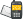It is currently 29 Oct 2020, 16:43### GMAT Club Daily Prep

#### Thank you for using the timer - this advanced tool can estimate your performance and suggest more practice questions. We have subscribed you to Daily Prep Questions via email.

Customized
for You

we will pick new questions that match your level based on your Timer History

Track

every week, we’ll send you an estimated GMAT score based on your performance

Practice
Pays

we will pick new questions that match your level based on your Timer History

#### Not interested in getting valuable practice questions and articles delivered to your email? No problem, unsubscribe here.# A polygon has 12 edges. How many different diagonals does itQuestion banks Downloads My Bookmarks Reviews Important topics
Author Message
TAGS:
Retired ModeratorJoined: 07 Jun 2014
Posts: 4803
GRE 1: Q167 V156WE: Business Development (Energy and Utilities)
Followers: 173

Kudos [?]: 2976 , given: 394

A polygon has 12 edges. How many different diagonals does it [#permalink]
Expert's post00:00

Question Stats:66% (00:58) correct33% (00:52) wrongbased on 42 sessions
A polygon has 12 edges. How many different diagonals does it have? (A diagonal is a line drawn from one vertex to any other vertex inside the given shape. This line cannot touch or cross any of the edges of the shape. For example, a triangle has zero diagonals and a rectangle has two.)

(A) 54
(B) 66
(C) 108
(D) 132
(E) 144
[Reveal] Spoiler: OA

_________________

Sandy
If you found this post useful, please let me know by pressing the Kudos Button

Try our free Online GRE Test

ManagerJoined: 27 Feb 2017
Posts: 188
Followers: 1

Kudos [?]: 78 , given: 15

Re: A polygon has 12 edges. How many different diagonals does it [#permalink]
someone please explain how to calculate this?Active MemberJoined: 07 Jan 2018
Posts: 694
Followers: 11

Kudos [?]: 778  , given: 88

Re: A polygon has 12 edges. How many different diagonals does it [#permalink]
1
KUDOS
Total number of diagonals of n sided polygon is given by the formula n(n-3)/2

Posted from my mobile device_________________

This is my response to the question and may be incorrect. Feel free to rectify any mistakes
Need Practice? 20 Free GRE Quant Tests available for free with 20 Kudos

Retired ModeratorJoined: 07 Jun 2014
Posts: 4803
GRE 1: Q167 V156WE: Business Development (Energy and Utilities)
Followers: 173

Kudos [?]: 2976 , given: 394

Re: A polygon has 12 edges. How many different diagonals does it [#permalink]
Expert's post
Explanation

A diagonal of a polygon is an internal line segment connecting any two unique vertices; this line segment does not lie along an edge of the given shape. Consider a polygon with 12 vertices.

Construct a diagonal by choosing any two vertices and connecting them with a line. Remember that this is order independent; the line is the same regardless of which is the starting vertex. Therefore, this is analogous to choosing any 2 elements from a set of 12, and can be written as $$\frac{12!}{10! \times 2!}= 6 \times 11 = 66$$.

However, this method includes the vertices connected to their adjacent vertices, which form edges instead of diagonals. In order to account for this, subtract the number of edges on the polygon from the above number: 66 – 12 = 54.

Alternatively, choose a random vertex of the 12-sided shape. There are 12 – 1 = 11 lines that can be drawn to other vertices since no line can be drawn from the vertex to itself. However, the lines from this vertex to the two adjacent vertices will lie along the edges of the polygon and therefore cannot be included as diagonals (see the figure of a pentagon below for an example):

Attachment:Capture.PNG [ 116.3 KiB | Viewed 4446 times ]

Thus, there are 12 – 1 – 2 = 9 diagonals for any given vertex. Since there are 12 vertices, it is tempting to think that the total number of diagonals is equal to (12)(9) = 108.

However, this scheme counts each diagonal twice, using each side of the diagonal once as the starting point. Therefore, there are half this many different diagonals: $$\frac{108}{2}= 54$$.
_________________

Sandy
If you found this post useful, please let me know by pressing the Kudos Button

Try our free Online GRE Test

InternJoined: 26 Oct 2018
Posts: 8
Followers: 0

Kudos [?]: 3 , given: 1

Re: A polygon has 12 edges. How many different diagonals does it [#permalink]
n(n-1)/2 - n
12(12-1)/2 - 12
66 - 12 = 54
ManagerJoined: 01 Nov 2018
Posts: 87
Followers: 0

Kudos [?]: 70 , given: 22

Re: A polygon has 12 edges. How many different diagonals does it [#permalink]
Expert's post
It's nice to know the diagonal formula, but in case you dont want to memorize the formula ( which will save you tons of time) then go about it this way..
a rectangle has 2 diagonals
a pentagon has 5 diagonals
a hexagon has 9 diagonals.
see the pattern?

the first increase of diagonals is from 0 to 2, then the next jump increases by 3 the next jump increases by 4, the next jump increases by 5.. and so on

diagonals 0 2 5 9 14 20 27 35 44 54
sides____ 3 4 5 6 7_ 8_ 9_ 10_ 11_ 12
_Re: A polygon has 12 edges. How many different diagonals does it   [#permalink] 01 Jan 2019, 23:28
Display posts from previous: Sort by

# A polygon has 12 edges. How many different diagonals does itQuestion banks Downloads My Bookmarks Reviews Important topicsPowered by phpBB © phpBB Group Kindly note that the GRE® test is a registered trademark of the Educational Testing Service®, and this site has neither been reviewed nor endorsed by ETS®.